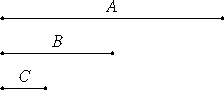Proposition 31

Any composite number is measured by some prime number.

Let A be a composite number.

I say that A is measured by some prime number.VII.Def.13

Since A is composite, therefore some number B measures it.

Now, if B is prime, then that which was proposed is done.

VII.Def.11,13

But if it is composite, some number measures it. Let a number C measure it.

Then, since C measures B, and B measures A, therefore C also measures A. And, if C is prime, then that which was proposed is done. But if it is composite, some number measures it. Thus, if the investigation is continued in this way, then some prime number will be found which measures the number before it, which also measures A. If it is not found, then an infinite sequence of numbers measures the number A, each of which is less than the other, which is impossible in numbers.

Therefore some prime number will be found which measures the one before it, which also measures A.

Therefore any composite number is measured by some prime number.

Therefore, any composite number is measured by some prime number.

Q.E.D.

Guide

In order to prove this proposition, Euclid again uses the unstated principle that any decreasing sequence of numbers is finite.

This proposition is used in the next one and in propositions IX.13 and IX.20.